## Sunday, February 1, 2009

### Irodov Problem 1.265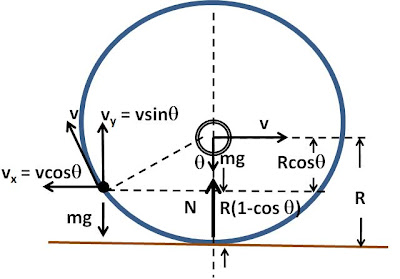As the point mass m attached to the hoop moves up it gains potential energy. Since total energy must be conserved, the kinetic energy of the (hoop+point mass system) reduces and thus the rotating hoop slows down. When the point mass m on the hoop is at an angle, say the linear velocity of the hoop is v. Since the hoop rolls without slipping, to an observer moving with the center of the hoop, the tangential velocity of the point mass will be v as shown in the figure. The net velocity of the point mass will thus be,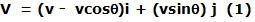The kinetic energy of the point mass is given by,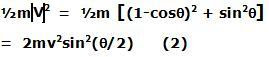The kinetic energy if the hoop is the sum of its translational and rotational kinetic energies given by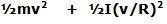where I is the moment of inertia of the hoop. Since every point of the hoop is at a distance of R from its axis of rotation passing though its center, its moment of inertia must be. Thus, the kinetic energy of the hoop is given byThe total kinetic energy of the hoop + point mass system is thus given by,As the point mass moves up as it moves with the rotating hoop, its potential energy increases. As seen in the figure, when the point mass is at an angleit is at a height of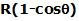. Thus its potential energy increases bycompared to when the point mass is at the ground level.

The total energy of the (hoop+point mass) system is the sum of kinetic and potential energies and is given by,When the point mass is at its lowest point the velocity of the hoop is vo and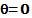and so the total energy of the (hoop+point mass system) when the point mass is at the lowest point using Eqn (4a) is given by. To physically understand what is happening at this point, the lowest point of the hoop is stationary since it is moving forward with a velocity vo with the hoop and backward with a velocity vo as it moves along the circumeference of the hoop and so its net velocity is 0. This at the lowest point, the point mass has no kinetic energy, and it has no potential energy since its at the ground. The total energy of the (hoop + point mass) system is only the kinetic energy of the hoop.

Now, since energy of the hoop is conserved at all points of time we have,Thus, now using (4b) we can find the linear velocity of the hoop as a function of- the angle at which the point mass lies on the hoop as,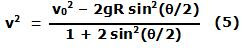From (5) we know the linear velocity of the hoop as an function of the angle of the point mass.

Now let us examine the forces acting on the (hoop + point mass) system. There are three forces acting on the system in the vertical direction, i) mg acting on the hoop,ii) mg acting on the point mass and iii) the normal reaction N from the floor. In other words the net vertical force of the (hoop + point mass) system is N-2mg.

The net sum of these forces must be equal to the rate of change of the vertical component of momentum of the CG of the (hoop + point mass) system. The vertical component of the momentum of the CG of the (hoop + point mass) system is the sum of two parts, i) the vertical component of momentum of the CG of the hoop and ii) the vertical component of the momentum of the point mass. The CG of the hoop (located at its center) has no vertical momentum since it moves in a horizontal straight line, thus this is 0. The attached point mass has vertical velocity of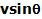and thus has a momentum of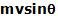. The net vertical momentum of the CG of the (hoop + point mass) system is thus equal to. Thus, we have,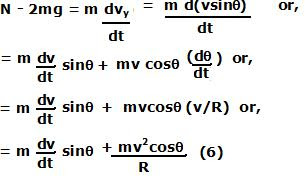In (6), essentially there are two source from where d(vy)/dt comes, i) the vertical component of the centripetal acceleration relative to the center of the hoop and ii) the retardation of the vertical component of point mass's velocity as the hoop's rotation slows down due to upward motion of the point mass. This is shown in the figure below:From (5) we have,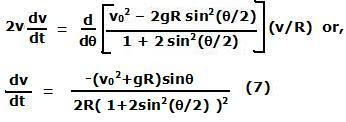When the hoop jumps up the normal reaction from the surface N=0 (since there is no contact with the surface). Thus, by setting N=0 in (6) we have,So substituting (7) and (5) in (7a) in we have,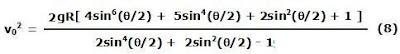Eqn (8) gives the value of velocity vo (when the point mass is at the bottom) at which the hoop will jump off of the surface when the point mass is at an angle. We however, need to find the minimum value of vo across all values ofwhich will cause the hoop to jump off.

Lets set, then we have,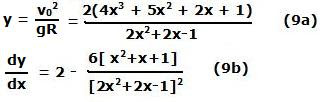Since only valid situations are for y>0 Equation (9a) has solutions only when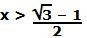(this corresponds to> 70.148 degrees). The derivative is provided in Eqn (9b). In the range( this corresponds to 70.148 <<180 degrees) the derivative dy/dx is negative. This means that the value of vo monotonically decreases in the rangeand attains it minima when the derivative becomes 0 at x=1 or= 180 degrees. In other words, the hoop will have its maximum tendency to jump off the ground when the point mass is located at the highest point.

Thus, settingin Eqn (8) we have